# Machine Learning Workflow using Pycaret

PyCaret is an open-source machine learning library which is simple and easy to use. It helps you right from the start of data preparation to till the end of model analysis and deployment. Moreover, it is essentially a python wrapper around several machine learning libraries and frameworks such as scikit-learn, spaCy etc, It also has the support of complex machine learning algorithms which are tedious to tune and implement.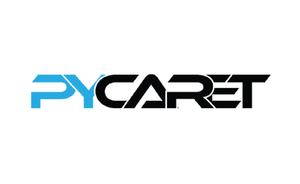So why to use Pycaret. Well, there are lots of reasons for this let me explain to you a few of them. The first Pycaret is a low-code library which makes you more productive while solving a business problem. Second Pycaret can do data preprocessing and feature engineering with a single line of code, where in reality, it is very time-consuming. Third Pycaret allows you to compare different machine learning models and finetune your model very easily. Well, there are many other advantages but for now, stick with them.

#### Installation

```pip install pycaret
```

if you are using Azure Notebooks or Google Colab

```!pip install pycaret
```

Let’s start by importing required libraries.

## Python3

 `# importing required libraries  ` `# for reading and manipulating data ` `import` `numpy as np ` `import` `pandas as pd`

Reading the dataset using pandas library

## Python3

 `# reading the data from csv file ` `iris_classification ``=` `pd.read_csv(``'Iris.csv'``)  ` ` `  `# viewing top 5 rows of data ` `iris_classification.head(``5``)`

Output: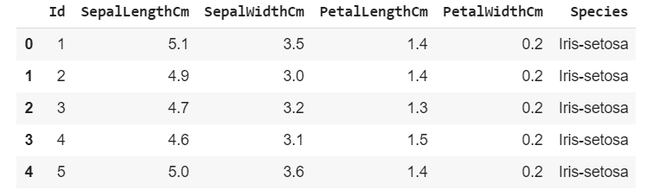## Starting with pycaret

Initializing the setup

## Python3

 `#import classification module from pycaret ` `from` `pycaret.classification ``import` `*` ` `  `#intialize the setup ` `clf ``=` `setup(iris_classification, target ``=` `'Species'``)`

setup takes our data iris_classification and the target value(which needs to predicted) in our case it is Species

Output: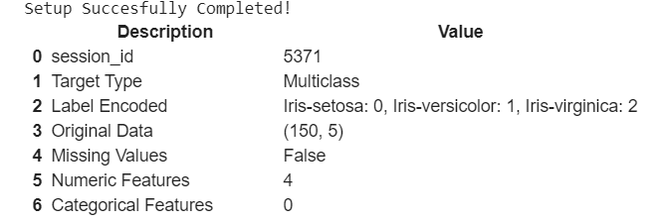It gives basic description of our dataset, you can see it automatically encoded the target variables into 0,1,2.

Now let’s compare various classification models that Pycaret built for us

## Python3

 `# comparing different  ` `# classification models ` `compare_models()`

Output: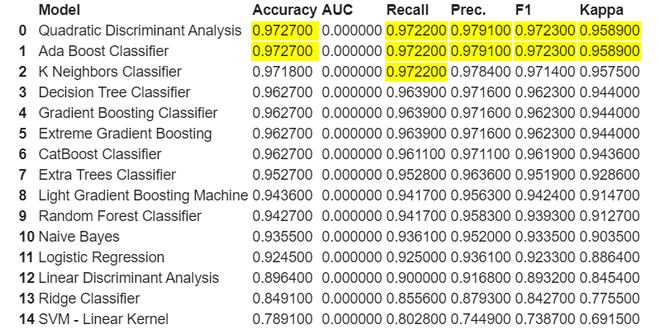As we can see here it highlights the highest value in each respective column. Here for this classification both Quadratic Discriminant Analysis and Ada Boost Classifier both are performing well let’s take QDA for our further model creation and analysis.

Creation of model

## Python3

 `# creating model qda ` `model ``=` `create_model(``'qda'``)`

Output: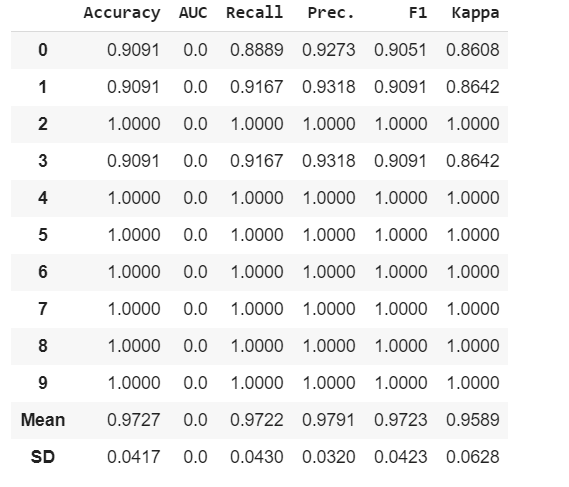It shows various metrics used to evaluate model on different folds.

Let’s tune the model hyperparameters

## Python3

 `# tuning model hyperparameters ` `tuned_model ``=` `tune_model(``'qda'``)`

Output: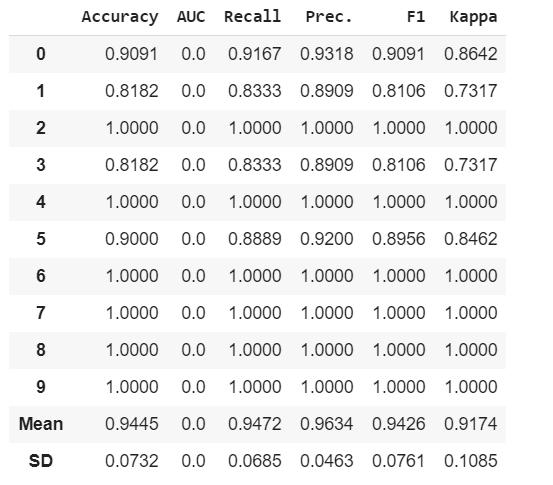We can see here some Recall, Precision, F1 and Kappa has increased because of fine tuning of our model.

Now let’s do some model analysis

## Python3

 `# plotting boundries between different ` `# labels ` `plot_model(tuned_model, plot ``=` `'boundary'``)`

Output: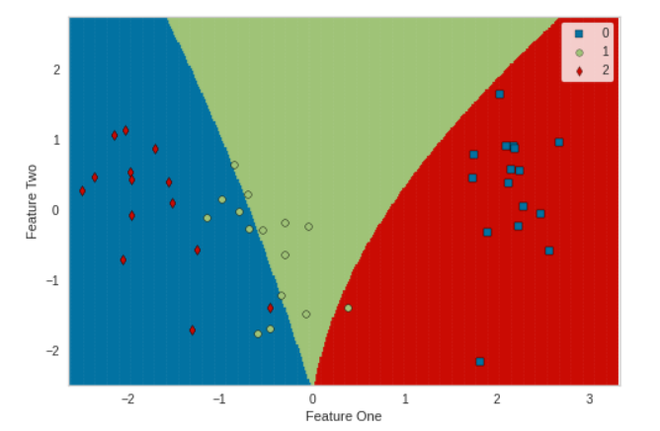## Python3

 `# plotting cnfusionmatrix for predicted labels ` `plot_model(tuned_model, plot ``=` `'confusion_matrix'``)`

Output: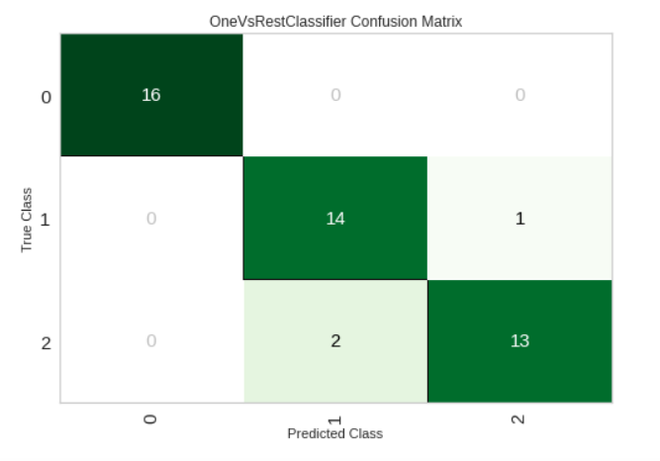## Python3

 `# plotting number of correctly  ` `# classified and misclassifed labels ` `plot_model(tuned_model, plot ``=` `'error'``)`

Output: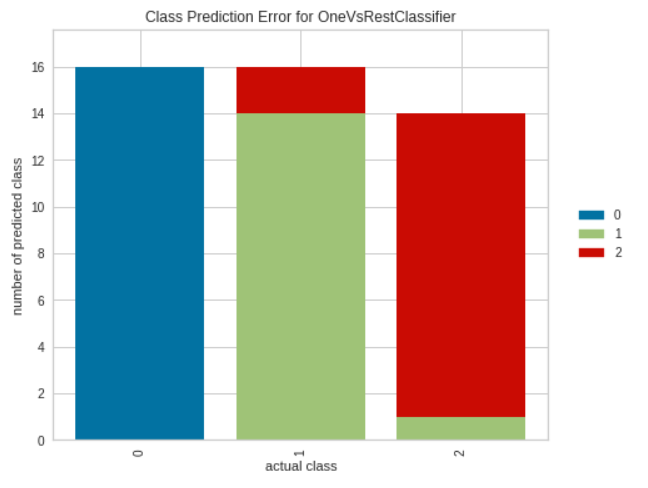## Python3

 `# plotting classification report ` `plot_model(tuned_model, plot ``=` `'class_report'``)`

Output: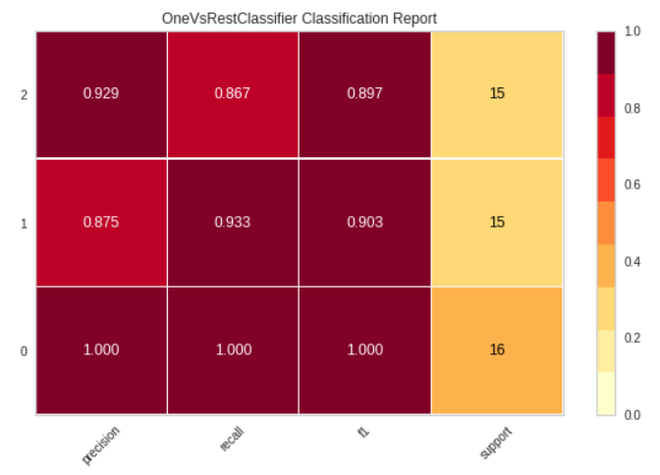Finalize the model

## Python3

 `# finalizing the tuned_model ` `finalize_model(tuned_model)`

Output: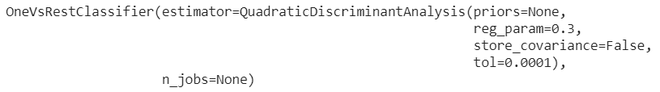Saving the model

## Python3

 `# saving the model ` `save_model(tuned_model, ``'qda1'``)`

Output: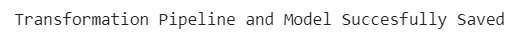My Personal Notes arrow_drop_upCheck out this Author's contributed articles.

If you like GeeksforGeeks and would like to contribute, you can also write an article using contribute.geeksforgeeks.org or mail your article to contribute@geeksforgeeks.org. See your article appearing on the GeeksforGeeks main page and help other Geeks.

Please Improve this article if you find anything incorrect by clicking on the "Improve Article" button below.